# Formula Basics

## Formula Basics in Google Sheets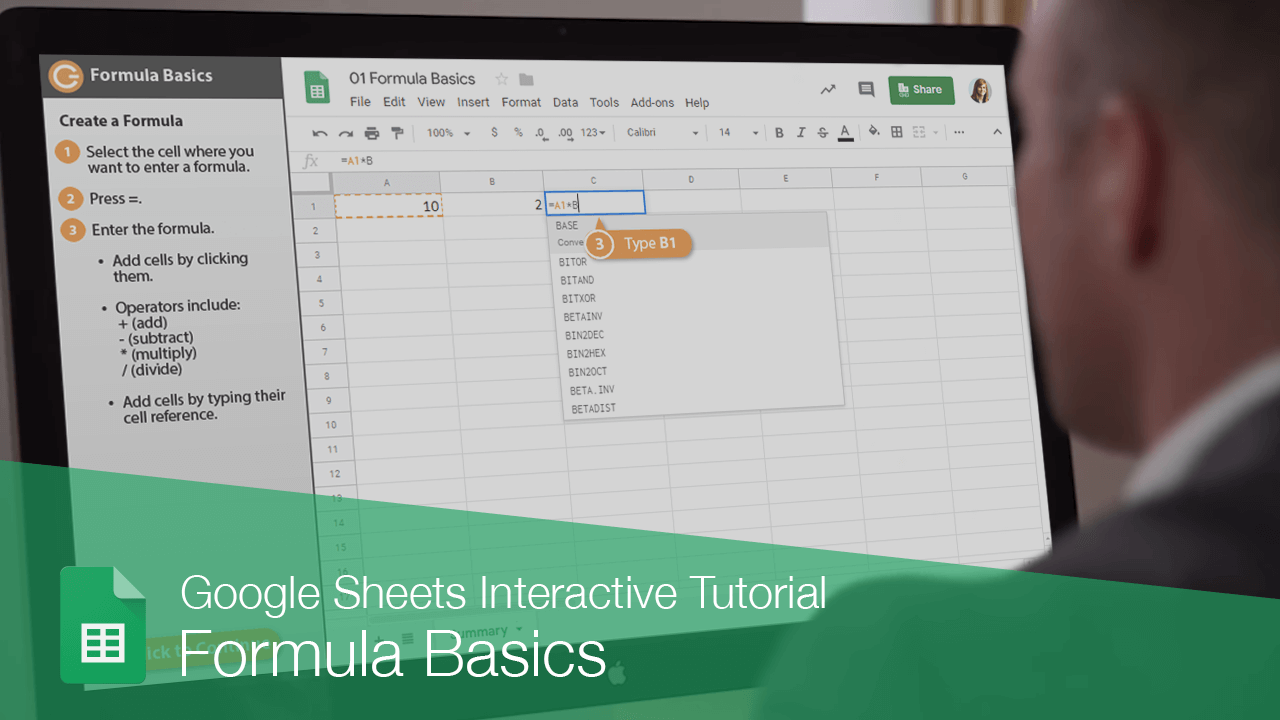Formulas contain information to perform a numerical calculation such as adding, subtracting, or multiplying. All formulas must start with an equal sign (=), followed by:

• Values or cell references joined by an operator. Example: =5+3 or =A1+A2.
• A function name followed by parentheses containing function arguments. Functions are the most common way to enter formulas in Sheets. Example: =SUM(A1:A2).

### Create a Formula

1. Select the cell where you want to enter a formula.
2. Press =.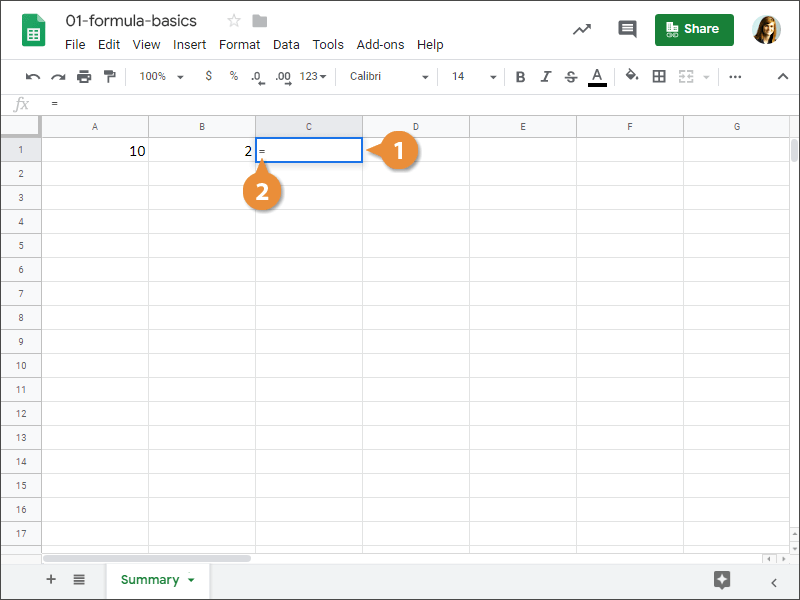3. Enter the formula.
• Add cells by clicking them or typing their cell reference.
• Operators include:

- (subtract)

* (multiply)

/ (divide)

4. Press Enter when you're finished.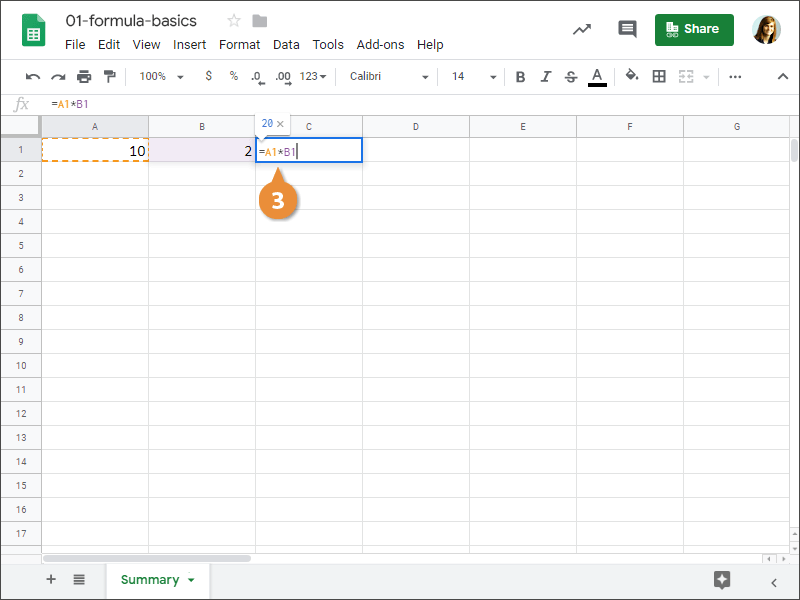If you entered the formula correctly, the results of the formula will appear in the cell.

Operator Formula Example Description
- =A1-B1 Subtracts values.
* =B1*2 Multiplies values.
/ =A1/C2 Divides values.
SUM =SUM(A1:A3) Totals numbers in a range.
AVERAGE =AVERAGE(A2,B1,C3) Averages numbers in a range.

### Update a Formula Result

Every time a cell that a formula references is updated, the formula result updates as well. Let's see that in action.

1. Formulas will automatically recalculate when changes are made to referenced cells.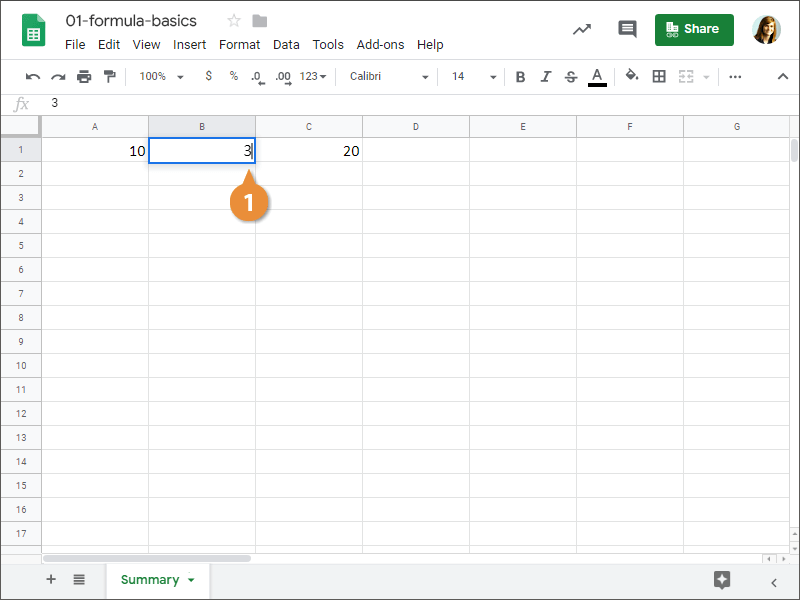### Edit a Formula

1. Select the cell where you want to edit a formula.
2. Click in the formula bar.3. Edit the formula in the formula bar.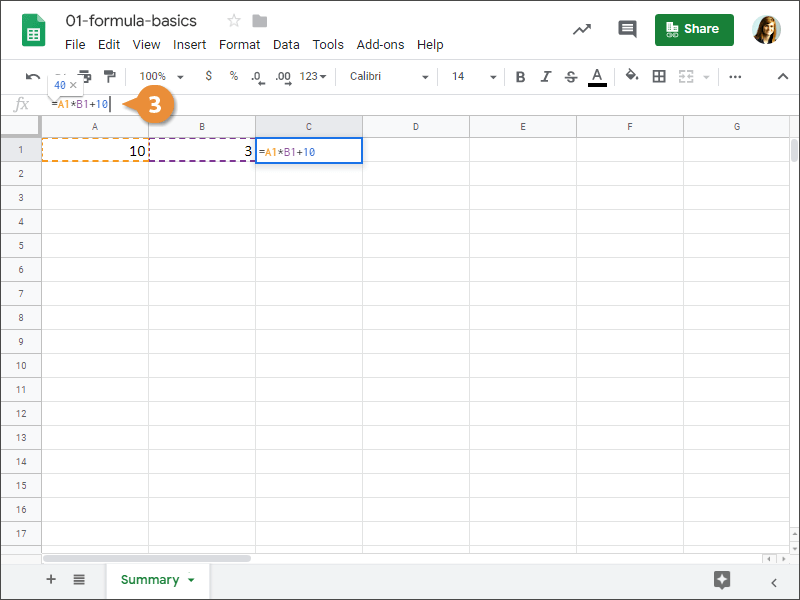4. Press Enter.

The formula is edited based on the changes you made to it.